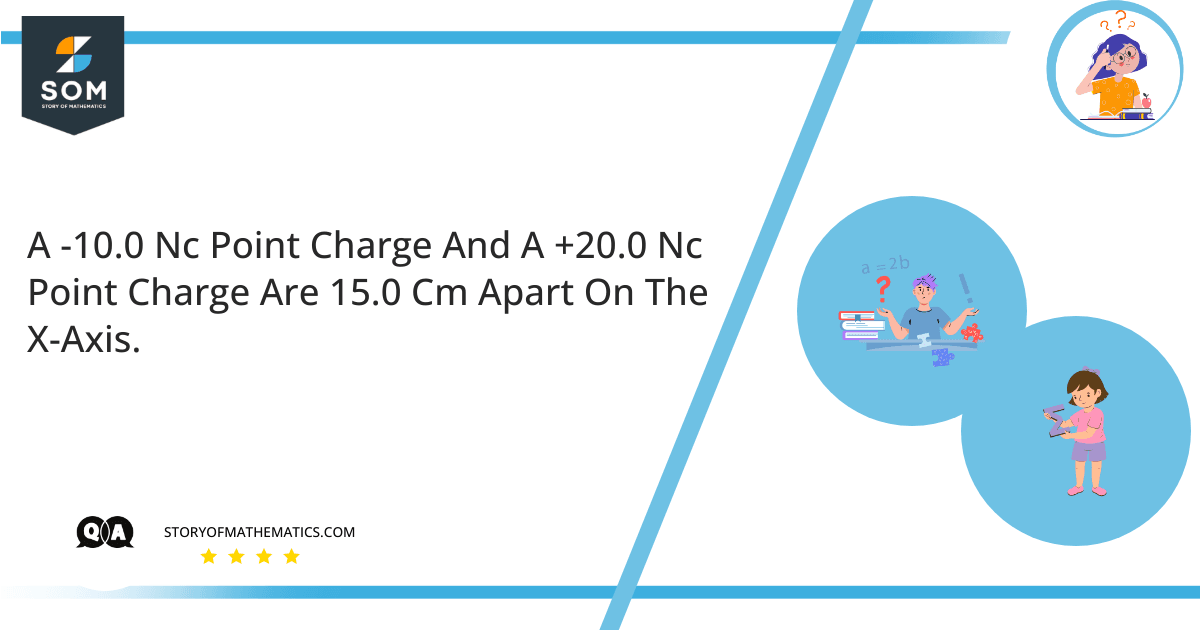# A -10.0 nC point charge and a +20.0 nC point charge are 15.0 cm apart on the x-axis. Find the following:• What is the electric potential at the point on the x-axis where the electric field is zero?
• What are the magnitude and direction of the electric field at the point on the x-axis, between the charges, where the electric potential is zero?
This question aims to find the electric potential at the point on x-axis where the electric field is zero. It also aims to find the magnitude and direction of the electric field where the electric potential is zero. This question is based on the concept of electric potential energy, which is defined as the work done for moving a charge from one point to another in the presence of an electric field. The electric field is defined as a field present around a charged particle in space and it will exert force on other charged particles if present in the same field. Coulomb’s Law can be used to find electric potential.

Two point charges $q_1$ and $q_2$ are present on the $x-axis$ with $-10 nC$ and $20 nC$, respectively. Assuming $q_1$ on the origin and $q_2$ is $15 cm$ apart from it, the electric potential due to two point charges is given as: $V = V_1 + V_2$ Where $V_1$ and $V_2$ are given as: $V_1 = k \dfrac{q_1}{r}$ $V_2 = k \dfrac{q_2}{15 – r}$Figure-1 : Depiction of Charges

a) We need to find the electric potential at the point on $x-axis$ where the electric field is zero. We can equate the potentials due to both point charges to get the point on $x-axis$.

$\dfrac{k |q_1|}{r^2} = \dfrac{k q_2}{(15 – r)^2}$

$\dfrac{|q_1|}{r^2} = \dfrac{q_2}{(15 – r)^2}$

$|q_1|(15 – r)^2 = q_2 r^2$

By substituting and solving the equation, we get:

$r = [6.21 cm , -36.21 cm]$

We know that at $r=6.21 cm$, the electric field can not be zero. So at $r=-36.21 cm$ the electric field is zero on the $x-axis$ as the point shown in Figure 2. Now to find the electric potential at this point, we need to substitute the values in the equation defined above, which is given as:

$V = k \dfrac{|q_1|}{r} + k \dfrac{q_2}{15 – r}$

Here $k$ is the constant and its value is given as:

$k = 9 \times 10^9 N.m^2/C^2$

Substituting the values of $q_1, q_2, k, \text{and} r$ we get:

$V = 9 \times 10^9 N.m^2/C^2 \big{[} \dfrac{10 \times 10^{-9}C}{-36.21 cm} + \dfrac{20 \times 10^{-9}C}{15 – (-36.21 cm)} \big{]}$

Simplifying the equation, we get:

$V = 103 V$

b) The point where the electric potential is zero can be calculated by the equation of electric potential by equating it to zero. The equation is given as:

$V = V_1 + V_2$

By putting $V=0$, we can find the point where the electric potential is zero between two oppositely charged point charges.

$0 = k \dfrac{q_1}{r} + k \dfrac{q_2}{15 – r}$

$– k \dfrac{q_1}{r} = k \dfrac{q_2}{15 – r}$

$– q_1(15 – r) = q_2 r$

$r = -15 (\dfrac{q_1}{q_2 – q_1})$

By substituting the values, we get:

$r = 5 cm$

Now we simply substitute the values in the equation to calculate the magnitude of electric field at $r=5 cm$. Equation is given as:

$E = E_1 + E_2$

$E = k \dfrac{|q_1|}{r^2} + k \dfrac{q_2}{(15 – r)^2}$

Substituting the values and solving the equation, we get:

$E = 54 \text{kV/m}$

The direction of the electric field will be in the direction of the vector sum of the given two point charges $\overrightarrow{E_1}$ and $\overrightarrow{E_2}$. The direction of the electric field will be from $q_2$ towards $q_1$, which is towards negative $x-axis$.

## Numerical Results:

a) The electric potential on the point where the electric field is zero on the $x=axis$ is:

$V = 103 V$

b) The magnitude of the electric field on the point where the electric potential is zero on $x-axis$ is:

$E = 54 \text{kV/m} \quad \text{Its direction will towards negative x-axis}$

## Example:

A $-5 \mu C$ point charge and a $5 \mu C$ point charge are $7 cm$ apart from each other. Find the electric field given by these point charges at the mid-point between these charges.Figure-2 : Point Charges

The electric field is given by,

$E = E_1 + E_2$

$E = k \Big{[} \dfrac{ 5 \times 10^{-6} C}{3.5 cm} + \dfrac{ 5 \times 10^{-6} C}{3.5 cm} \Big{]}$

$E = 9 \times 10^{9} Nm^2/C^2 \Big{[} \dfrac{ 5 \times 10^{-6} C}{3.5 cm} + \dfrac{ 5 \times 10^{-6} C}{3.5 cm} \Big{]}$

By solving it, we get:

$E = 2.6 \times 10^6 N/C$

Images/Mathematical drawings are created with Geogebra.